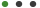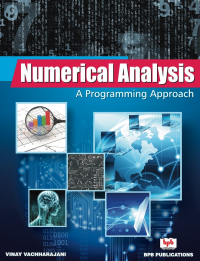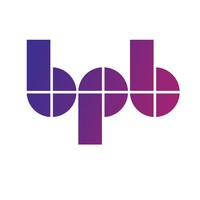# Numerical Analysis

Learn the concept of algorithms from beginners to advanced levelVinay VachharajaniBPB PublicationsLanguage - EnglishUpdated on Mar, 2021Teaching & Academics,MathThis eBook includes

Formats : PDF (Downlodable)

Pages : 596

ISBN : 9788183335515

Language : English

### Book description

Learn the concept of algorithms from beginners to advanced level

## Key Features

â— Chapters include objectives, learning outcomes, multiple choice questions, exercises for practice and solutions.
â— Programs are written in C Language for Numerical methods.
â— Topics are explained with suitable examples.
â— Arrangement (Logical order), clarity, detailed presentation and explanation of each topic with numerous solved and unsolved examples.
â— Concise but lucid and student friendly presentation for derivation of formulas used in various numerical methods.

## Description

In the present scenario where the knowledge of computers has become an absolute necessity, there has been an increasing desire for numerical answers to any applied problem. This has led to high demand for courses in numerical analysis. Many real world problems are solved first by converting them into mathematical models and then applying analytical methods to solve that model. In several cases, where ordinary analytical methods fail, numerical methods can give the result.
The book is divided into twelve chapters, describing the concept of computer arithmetic, errors, iterative methods to find the roots of transcendental and algebraic equations, curve fitting, numerical differentiation, integration, and so on.
In the book the major emphasis is given on numerous solved and unsolved examples. Many topics in this book such as process graph, cubic spline method, Taylor series, Legendre and Chebyshev polynomials, Gauss - Chebyshev integration method and boundary value problems make this book unique.

## What will you learn

â— Concept of numbers and their accuracy, binary and decimal number system, limitations of floating point representation.
â— Concept of error and their types, propagation of errors through process graph.
â— Iterative methods for finding the roots of algebraic and transcendental equations with their convergence, methods to solve the set of nonlinear equations, methods to obtain complex roots.
â— Concept of matrices, the direct and iterative methods to solve a system of linear algebraic equations.
â— Finite differences, interpolation and extrapolation methods, cubic spline, concept of curve fitting.
â— Differentiation and integration methods.
â— Solution of ordinary and partial differential equations

## Who this book is for

This book is designed to serve as a textbook for the undergraduate as well as post graduate students of Mathematics, Engineering, Computer science (MCA, BCA), Information Technology and M.Sc. (IT).

1. Computer Arithmetic
2. Error Analysis
3. Solution of Algebraic and Transcendental Equations
4. Solution of System of Linear Equations and Eigen value Problems
5. Finite Differences
6. Interpolation
7. Curve Fitting and Approximation
8. Numerical Differentiation
9. Numerical Integration
10. Difference Equations
11. Numerical Solution of Ordinary Differential Equations
12. Numerical Solution of Partial Differential Equations## Author DetailsBPB Publications

BPB is Asia's largest publishers of Computer & IT books. For the last 63 years BPB has been a friend, philosopher and guide for programmers, developers, hardware technicians, IT Professionals who have made things happen in the IT World.